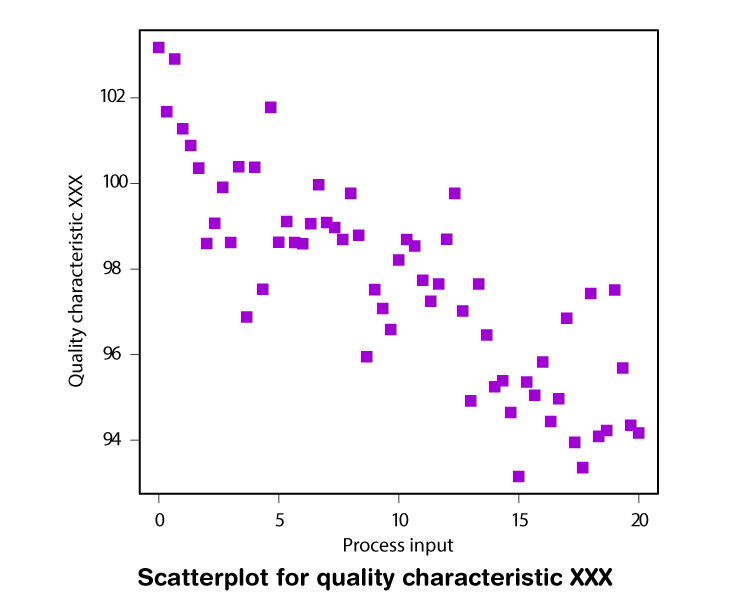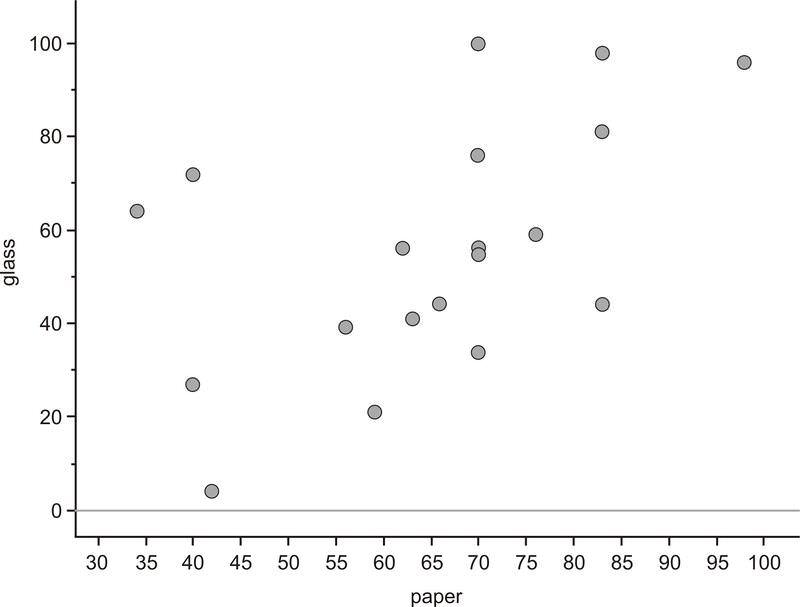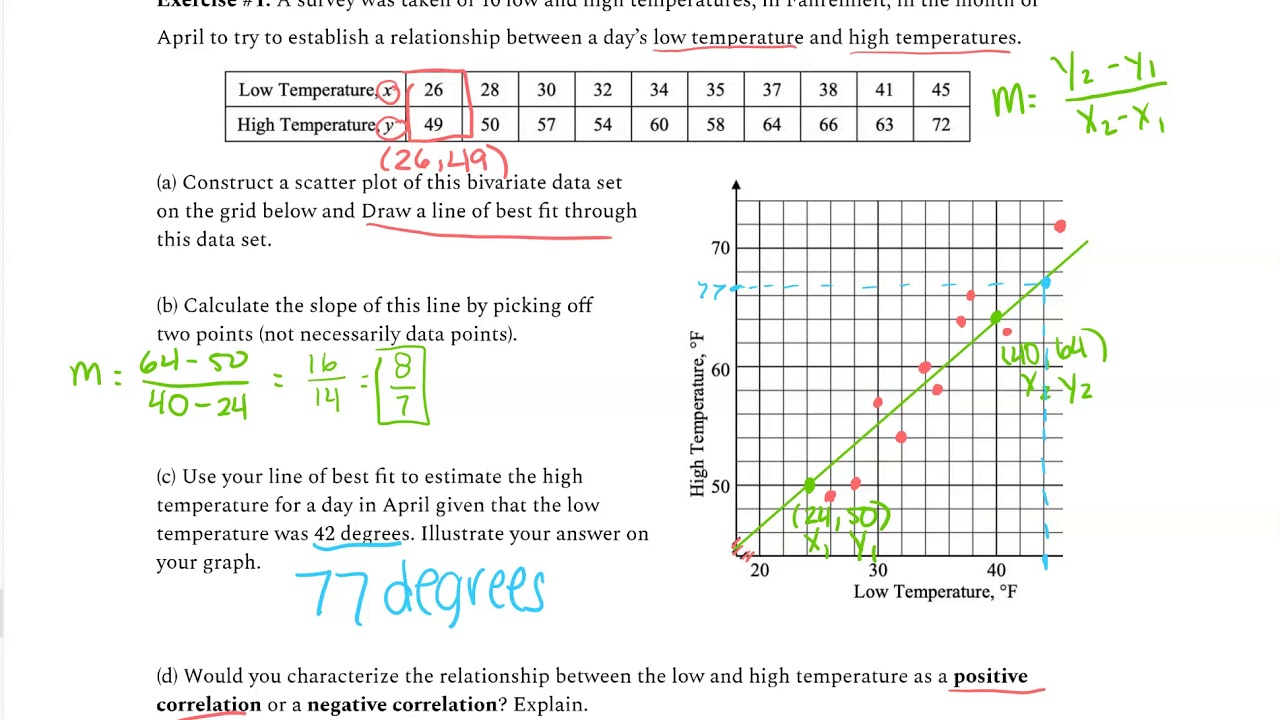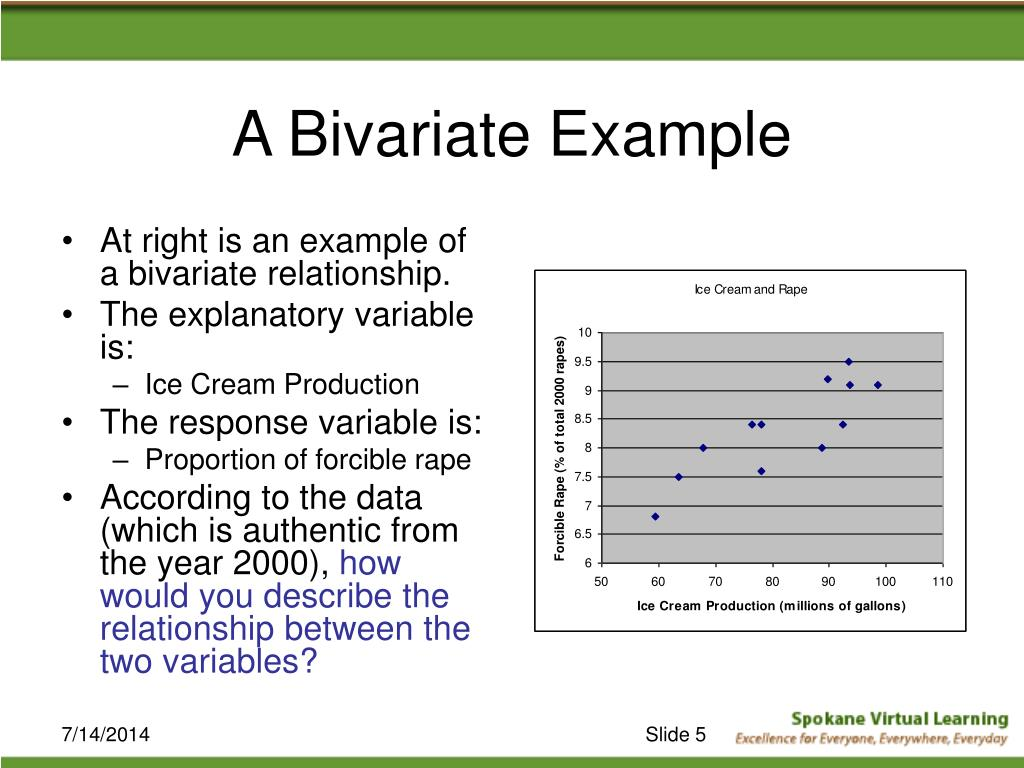## Bivariate Data: Examples, Definition and Analysis

• What is bivariate data? Definition.
• Examples of bivariate data: with table.
• Bivariate data analysis examples: including linear regression analysis, correlation (relationship), distribution, and scatter plot.

Let’s define bivariate data:

We have bivariate data when we studying two variables . These variables are changing and are compared to find the relationships between them.

For example, if you are studying a group of students to find out their average math score and their age, you have two variables (math score and age).

If you are studying only one variable, for example only math score for these students, then we have univariate data .

When we are examining bivariate data, the two variables could depend on each other. One variable could influence another. In this case, we say that the bivariate data has:

• an independent variable and
• a dependent variable .

A classical example of dependent and independent variables are age and heights of the babies and toddlers. When age increases, the height also increases.

Let’s move on to some real-life and practical bivariate data examples.

Look at the following bivariate data table. It represents the age and average height of a group of babies and kids.

Commonly, bivariate data is stored in a table with two columns.

There are 2 types of relationship between the dependent and independent variable:

• A positive relationship  (also called positive correlation) – that means if the independent variable increases, then the dependent variable would also increase and vice versa. The above example about the kids’ age and height is a classical positive relationship.
• A negative relationship (negative correlation) – when the independent variable increases and the dependent variable decrease and vice versa. Example: when the car age increases, the car price decreases.

So, we use bivariate data to compare two sets of data and to discover any relationships between them.

Bivariate Data Analysis

Bivariate analysis allows you to study the relationship between 2 variables and has many practical uses in the real life. It aims to find out whether there exists an association between the variables and what is its strength.

Bivariate analysis also allows you to test a hypothesis of association and causality. It also helps you to predict the values of a dependent variable based on the changes of an independent variable.

Let’s see how the bivariate data work with linear regression models .

Let’s say you have to study the relationship between the age and the systolic blood pressure in a company. You have a sample of 10 workers aged thirty to fifty-five years. The results are presented in the following bivariate data table.

Now, we need to display this table graphically to be able to make some conclusions.

Bivariate data is most often displayed using a scatter plot. This is a plot on a grid paper of y (y-axis) against x (x-axis) and indicates the behavior of given data sets.

Scatter plot is one of the popular types of graphs that give us a much more clear picture of a possible relationship between the variables.

Let’s build our Scatter Plot based on the table above:

The above scatter plot illustrates that the values seem to group around a straight line i.e it shows that there is a possible linear relationship between the age and systolic blood pressure.

You can create scatter plots very easily with a variety of free graphing software available online.

What does this graph show us?

It is obvious that there is a relationship between age and blood pressure and moreover this relationship is positive (i.e. we have positive correlation). The older the age, the higher the systolic blood pressure.

The line that you see in the graph is called “line of best fit” (or the regression line). The line of best fit aims to answer the question whether these two variables correlate. It can be used to help you determine trends within the data sets.

Furthermore, the line of best fit illustrates the strength of the correlation .

Let’s investigate further.

We constated that in our example, there is a positive and strong linear relationship between age and blood pressure. However, how strong is that relationship? What is its strength?

This is where the correlation coefficient comes to answer this question.

The correlation coefficient (R) is a numerical value measured between -1 and 1 . It indicates the strength of the linear relationship between two given variables. For describing a linear regression, the coefficient is called Pearson’s correlation coefficient.

When the correlation coefficient is closer to 1 it shows a strong positive relationship. When it is close to -1, there is a strong negative relationship. A value of 0 tells us that there is no relationship.

We need to calculate our correlation coefficient between age and blood pressure. There is a long formula (for Pearson’s correlation coefficient) for this but you don’t need to remember it.

All you need to do is to use a free or premium calculator such as those on www.socscistatistics.com  . When we put our bivariate data on this calculator we got the following result:

The value of the correlation coefficient (R) is 0.8435. It shows a strong positive correlation.

Now, let’s calculate the equation of the regression line (the best fit line) to find out the slope of the line.

For that purpose let’s remind the simple linear regression equation :

Y = Β 0  + Β 1 X

X  – the value of the independent variable, Y  – the value of the dependent variable. Β 0  – is a constant (shows the value of Y when the value of X=0) Β 1  – the regression coefficient (shows how much Y changes for each unit change in X)

Again, we will use the same online software ( socscistatistics.com ) to calculate the linear regression equation. The result is:

Y = 1.612*X + 74.35

More on linear regression equation and explanation, you can see in our post for linear regression examples .

So, from the above bivariate data analysis example that includes workers of the company, we can say that blood pressure increased as the age increased. This indicates that age is a significant factor that influences the change of blood pressure.

Other popular positive bivariate data correlation examples are: temperature and the amount of the ice cream sales, alcohol consumption and cholesterol levels, weights and heights of college students, and etc.

Let’s see bivariate data analysis examples for a negative correlation.

The below bivariate data table shows the number of student absences and their final grades in a class.

It is quite obvious that these two variables have a negative correlation between them.

When the number of student absences increases, the final grades decrease.

Now, let’s plot the bivariate data from the table on a scatter plot and to create the best-fit line:

Note how the regression line looks – it has a downward slope.

This downward slope indicates there is a negative linear association.

We can calculate the correlation coefficient and linear regression equation. Here are the results:

• The value of the correlation coefficient (R) is -0.9061 . This is a strong negative correlation.
• The linear regression equation is Y = -3.971*X + 90.71.

We can conclude that the least number of lessons the students skip, the higher grade could be reached.

Conclusion:

The above bivariate data examples aim to help you understand better how does the bivariate analysis work.

Analyzing two variables is a common part of the inferential statistics types and calculations. Many business and scientific investigations include only two continuous variables.

The main questions that bivariate analysis has to answer are:

• Is there a correlation between 2 given variables?
• Is the relationship positive or negative?
• What is the degree of the correlation? Is it strong or weak?

If you need other practical examples in the area of management and analysis, our posts Venn diagram examples and decision tree examples might be helpful for you.## Silvia Valcheva

Silvia Valcheva is a digital marketer with over a decade of experience creating content for the tech industry. She has a strong passion for writing about emerging software and technologies such as big data, AI (Artificial Intelligence), IoT (Internet of Things), process automation, etc.EXCELLENT and illustrative presentationExcellent. Simple and effective explanationsThanks. Happy to help!Clears the Whole concept in very simple and easy way,thanks a lot

This site uses Akismet to reduce spam. Learn how your comment data is processed .#### IMAGES

1. Bivariate Analysis- Definition and Types2. Displaying Bivariate Data3. The result of bivariate analysis4. Algebra I: Bivariate Data Analysis5. PPT6. Bivariate analysis| How to analyze data using spss (part-10)#### VIDEO

1. Complex Q3: Bivariate Data Analysis

2. (STAT101) CH3 And (STAT107)CH4 , Describing bivariate data

3. Data analysis part 1

4. StatCrunch Tutorial bivariate data analysis

5. IMG 1433

6. MS4 Bivariate Data Analysis 4 Line of Best Fit

1. Bivariate Analysis Definition & Example

Bivariate analysis means the analysis of bivariate data. It is one of the simplest forms of statistical analysis, used to find out if there is a relationship between two sets of values. It usually involves the variables X and Y. Univariate analysis is the analysis of one (“uni”) variable. Bivariate analysis is the analysis of exactly two variables.

2. 12 Bivariate Data Analysis: Regression and Correlation Methods

12.2 Graphical Representation of Bivariate Data A standard plot on a grid paper of y (y-axis) against x (x-axis) gives a very good indication of the behaviour of data. This coordinate plot of the points (x1;y1), (x2;y2);:::; (xn;yn) on a grid paper is called a scatter plot. Note: The ﬁrst step of the analysis of bivariate data is to plot the ...

3. Session 7 Bivariate Data and Analysis

data analysis, known as bivariate analysis, explores the concept of association between two variables. Association is based on how two variables simultaneously change together—the notion of co-variation. Learning Objectives The goal of this lesson is to understand the concepts of association and co-variation between two quantitative variables.

4. Chapter 22: Bivariate Analysis Assignment. Two Sample

Question: Chapter 22: Bivariate Analysis Assignment. Two Sample T-Test Attached Files: auto data.xls (20.5 KB) Research Analysis Assignment Outline.docx A (15.206 KB) Using the Auto Data set, which describes a set of vehicles and their prices in the 1970s, use a bivariate model to work through the Business Research Process using the attached outline document to

5. Bivariate Data Analysis: Examples, Definition, Data Sets

Bivariate analysis is a statistical method that helps you study relationships (correlation) between data sets. Many businesses, marketing, and social science questions and problems could be solved using bivariate data sets. On this page: What is bivariate data? Definition. Examples of bivariate data: with table.

6. Chapter 22: Bivariate Analysis Assignment. Two Sample

Expert Answer. Transcribed image text: Chapter 22: Bivariate Analysis Assignment. Two Sample T-Test Attached Files: auto_data.xls (20.5 KB) Research Analysis Assignment Outline.docx A (15.206 KB) Using the Auto Data set, which describes a set of vehicles and their prices in the 1970s, use a bivariate model to work through the Business Research ...